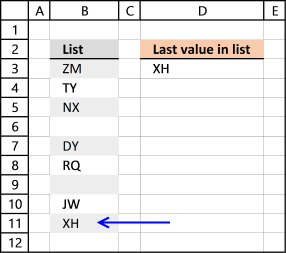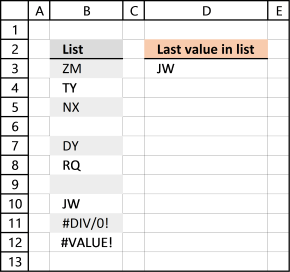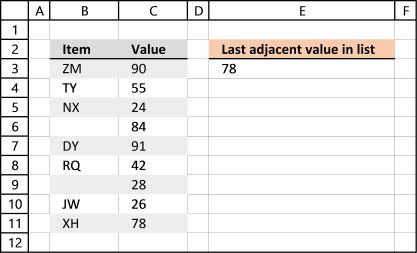Author: Oscar Cronquist Article last updated on October 17, 2018

The formula in cell D3 lets you get the last value in column B, it works fine with blank cells in your list.Formula in cell D3:

=LOOKUP(2,1/(B:B<>""),B:B)

The formula is quite cpu-intensive since it is processing all cells in column B, there are more than a million cells in one column in Excel 2007 and later versions.

Recommended article

Find last matching value in an unsorted list

I read an interesting blog post Find Last Item in Group With Index Match written by Debra Dalgleish. It is about […]

You can change the cell references if you know you will have a smaller list, this formula is easier for Excel to process:

=LOOKUP(2,1/(B1:B10000<>""),B1:B10000)

### Explaining formula

The formula takes all cell values in column B and checks if they are not empty. Then it divides 1 with the array creating errors for all empty cells.

The LOOKUP function ignores errors and tries to find a match. If every match is 1 and the lookup value is 2, the LOOKUP function returns last value in cell range. Normally the list must be sorted ascending for the LOOKUP function to work, however since every value is 1 there is no need to sort the list.

I am going to use the following formula because it will be easier to demonstrate:

LOOKUP(2,1/(B3:B12<>""),B3:B12)

Step 1 - Check if cells in column B are not equal to nothing

B3:B12<>""

becomes

{"ZM";"TY";"NX";0; "DY";"RQ";0; "JW";"XH";0}<>""

and returns

{TRUE;TRUE;TRUE; FALSE;TRUE;TRUE;FALSE; TRUE;TRUE;FALSE}

Step 2 - Divide 1 with array

1/(B3:B12<>"")

becomes

1/({TRUE;TRUE;TRUE; FALSE;TRUE;TRUE;FALSE; TRUE;TRUE;FALSE})

and returns

{1;1;1;#DIV/0!;1;1; #DIV/0!;1;1;#DIV/0!}

Boolean value TRUE is equal to 1 and FALSE is equal to 0. You can't divide a value with zero so Excel returns an error (#DIV/0!).

Step 3 - Return value

LOOKUP(2,1/(B3:B12<>""),B3:B12)

becomes

LOOKUP(2,{1;1;1;#DIV/0!;1;1; #DIV/0!;1;1;#DIV/0!},B3:B12)

becomes

LOOKUP(2,{1;1;1;#DIV/0!;1;1; #DIV/0!;1;1;#DIV/0!},{"ZM";"TY";"NX";0; "DY";"RQ";0; "JW";"XH";0})

and returns XH in cell D3.

Recommended article

How to use the LOOKUP function

Finds a value in a sorted cell range and returns a value on the same row.

I have added a few errors in column B below.I am working with Excel 2016 and errors in column B seems to not be an issue.

This data set has two columns, column B and C.Formula in cell E3:

=LOOKUP(2,1/(B:B<>""),C:C)

The formula returns an adjacent value of the last value. In fact, it doesn't need to be adjacent, you can change the cell reference (C:C) as long as it's starting point and ending point is the same as the first cell reference (B:B).